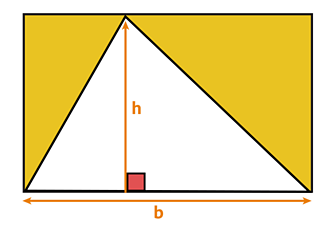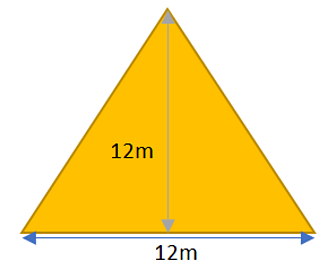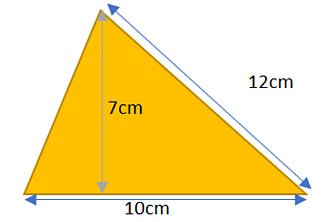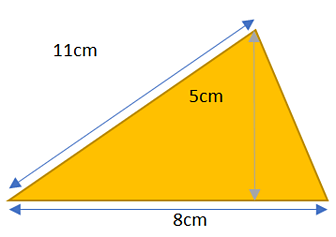# Learn

If you know how to calculate the area of a rectangle (base x height), you can find the area of a triangle with no problems!

The area of a triangle is always half the area of a rectangle that has the same height and width.

This is because a triangle can always fit into a rectangle twice, no matter the shape of the triangle.

So the formula we could use to find the area of a triangle is:

(base x height) ÷ 2

You can also write the formula as:

½ x base x height

They both give you the exact same answer since multiplying by a half is the same as dividing by 2.### Example 1:Use the formula ½ x base x height to find the area of the triangle above.

First let's multiply the measurements for the base and the height together:

12 x 12 = 144

Now let's multiply that by ½:

144 x ½ = 72 m²

So the area of the triangle is 72 m².

### Example 2:The height of this triangle is 7 cm and the base is 10 cm.

Always be careful that you are looking at the correct measurement for the height - the longest side of the triangle is not the same as the height!

Now use the formula (base x height) ÷ 2 to find the area of the triangle.

First let's multiply the base and the height:

7 x 10 = 70 cm

Now divide by 2:

70 ÷ 2 = 35 cm²

The area of the triangle is 35 cm².

Example 3:The height of this triangle is 5 cm and the base is 8 cm.

Use the formula (base x height) ÷ 2 to find the area of the triangle:

5 x 8 = 40 cm (base x height)

40 ÷ 2 = 20 cm²

The area of the triangle is 20 cm².

## Practise

Further your understanding and have a go at working out the area of some more triangles with this interactive guide from My Maths, University Oxford Press.

__________________________________________________________________________________________________________________

# Learn

Evolution is the way that living things change over time.

The first person who explained how evolution happens was Charles Darwin with his scientific theory of natural selection.

Charles Darwin observed that although individuals in a species shared similarities, they were not exact copies of each other; there were small differences or variations between them. He also noticed that everything in the natural world was in competition.

The winners were those that had characteristics which made them better adapted for survival. For example, they were stronger, faster, cleverer or more attractive than others in their species. These living things were more likely to reproduce and pass on their useful characteristics to their offspring.

Individuals that were poorly adapted were less likely to survive and their characteristics were not as likely to be inherited.

Over time, the characteristics that help survival become more common and a species gradually changes. Given enough time, these small changes can add up to the extent that a new species altogether can evolve.

## Charles Darwin

Charles Darwin published his scientific theory of natural selection in a book called ‘On the Origin of Species’ in 1859.

Darwin’s theory explained how every living thing is connected in a family tree that stretches back billions of years to the beginning of life on Earth.

## Glossary

• Adaptation - How living things are specialised to suit their environment.
• Evolution - The process by which living things can gradually change over time.
• Inheritance - The process of passing on features from parents to offspring.
• Species - A group of living things with very similar characteristics. They can breed together to make more living things of the same type.
• Variation - The differences between living things in a species.

Activity 1

Evolution Wordsearch - Any words you don't know, look them up and make a glossary.

Activity 2

Charles Darwin Research

__________________________________________________________________________________________________________________

Have a lovely weekend!

pflood@sfdprimary.co.uk